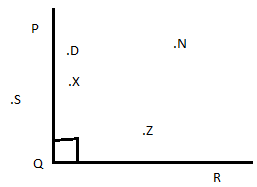1) Match the items in column I with those in column II

 Column I Column II 1) A three sided polygon a) diameter 2) The distance around a circle b) chord 3) Line segment passing through the centre of a circle c) angle 4) Every circle has a point at the d) circumference 5) Two rays starting from a common end points e) triangle f) centre

Ans: 1) e     2) d      3) a      4) f      5) c

2) State whether true or false: If false, correct the statement.
a) A line segment is a part of plane
b) Two lines in a plane always intersect each other in a point
c) Diameter of the circle divides it into two semi  circles
d) Every chord of a circle passes through the centre
e) A point indicates a definite position.
f) A line consist of finite number of points

Ans:
a) False: A line segment is a part of a line that has two end points

b) True
c) True
d) False: Diameter is the only chord that passes through the centre
e) True
f) False: A line consists of infinite number of points

3) Find the measure of the angel between hour and minute hands of a clock at 3’clock
Ans: The angle measure is 90

4) Answer the following questions based on the figure:
Ans: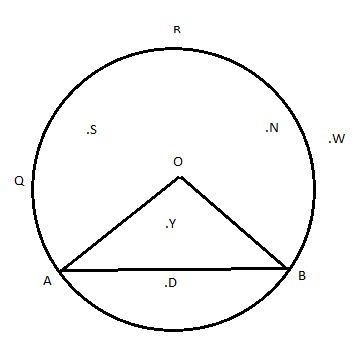Write points which are:
a) in the minor arc AXB                    b) in the minor segment AXB
c) in the major arc ARB                    d) major sector ARB
e) minor sector AXB
Ans: a) Y, D       b) D                 c) S, N, O            d) S, N, O           e) Y, D

5) Illustrate each of the following if possible with a rough diagram
a) A closed curve that is not a polygon
b) An open curve made up entirely of line segments
c) A polygon with two sides

Ans:
a)
b)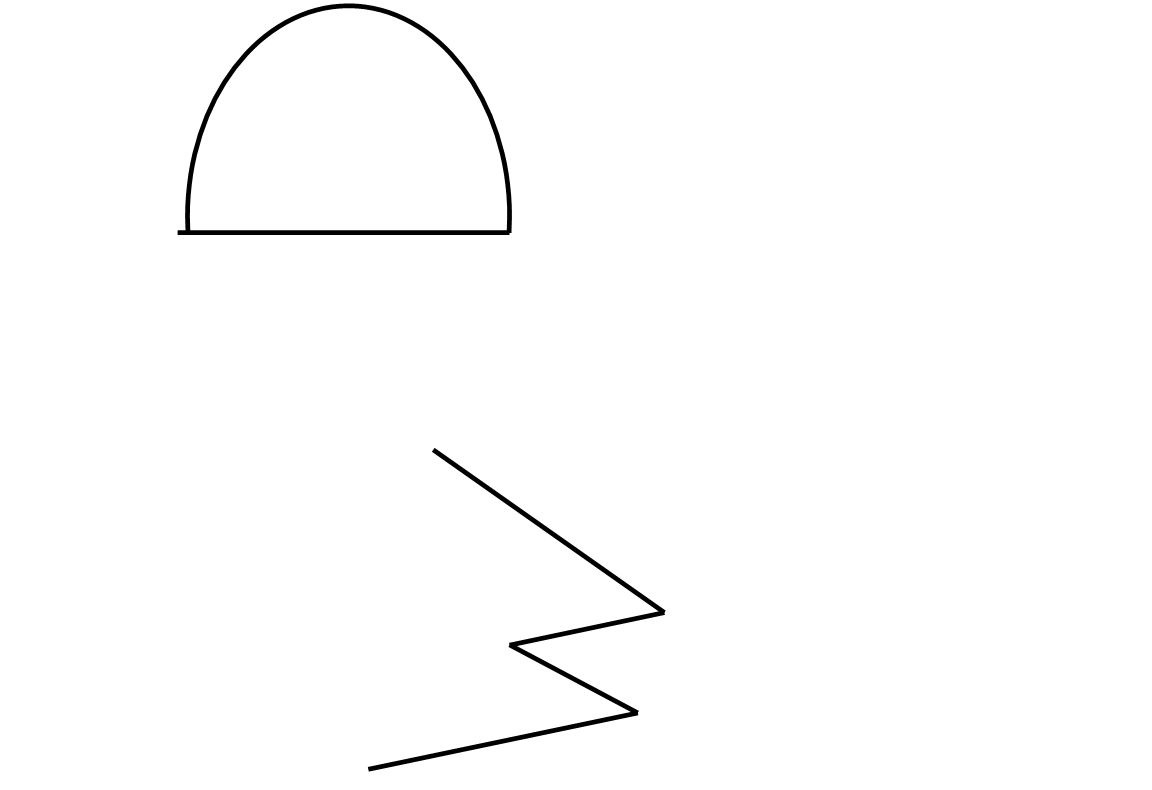c) Not possible

6) From the below given figure identify: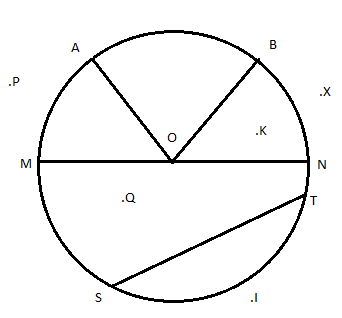a) segment     b) diameter       c) arc     d) two radii       e) centre of the circle

f) a chord       g) points in the exterior    h)  points in the exterior

Ans: a) ST     b) MN  c) SIT    d) OA, OB    e) O   f) ST        g) X, P       h) K, Q, O

7) From the figure of quadrilateral RICE identify the following and state:a) opposite sides     b) adjacent sides        c) diagonals
d) 2 pairs of adjacent angles         e) 2 pairs of opposite angles
Ans: a) RI, EC and RE, IC
b) RI, RE;    RI, IC;     IC, CE;     CE, ER
c) RC and IE
d) ∠ECR, ∠ICR and ∠ERC, ∠IRC

8) Classify the following as
a) Closed curve b) Open curves
a)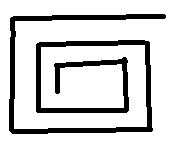b)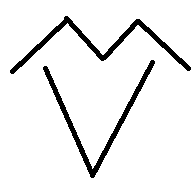c)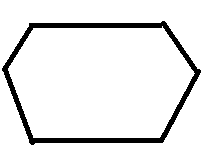d)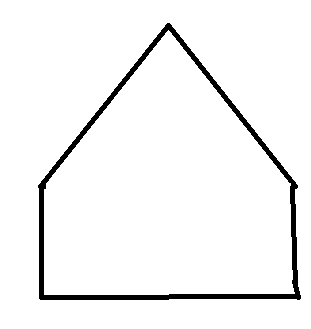e)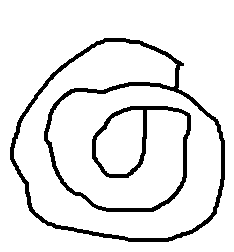d)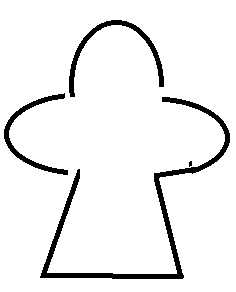e)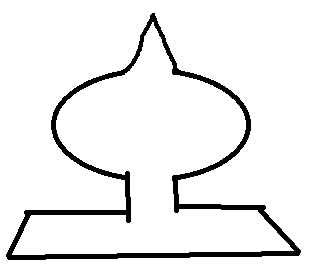a) c, e, g                     b) b, a, d, f

9) In the given diagram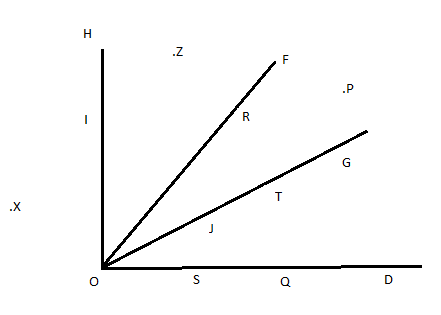i) Name point(s)
a) in the exterior of ∠HOD
b) in the interior of ∠FOD
c) on ∠FOD
ii) name three angles